Function Repository Resource:

# FocalSet

Compute the focal set of a surface

Contributed by: Wolfram Staff (original content by Alfred Gray)
 ResourceFunction["FocalSet"][i,s,{u,v}] gives a parametrization of the ith focal set of a surface s parametrized by u and v.

## Details and Options

i must be either 1 or 2.
The ith focal set of a surface s parametrized by u and v is defined as zi(u,v)=s(u,v)+U(u,v)/ki, where ki is the ith principal curvature of s and U is its unit normal.
The two focal sets can be two surfaces, a curve and a surface or two curves.

## Examples

### Basic Examples (2)

Define a hyperbolic paraboloid:

 In:=The focal sets of the hyperbolic paraboloid are given by:

 In:=Out=Plot an hyperbolic paraboloid (yellow) and its focal sets (blue and green):

 In:=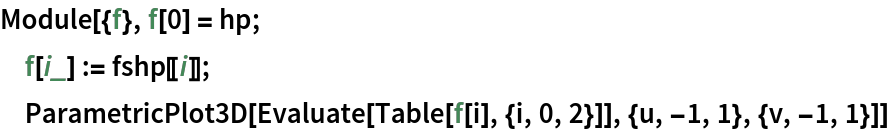Out=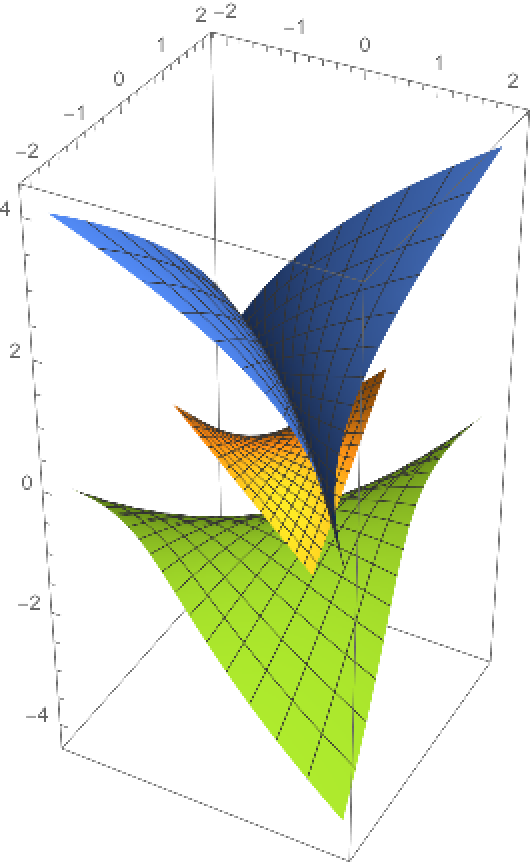Define an elliptic paraboloid:

 In:=The focal sets of an elliptic paraboloid are given by:

 In:=Out=Plot an elliptic paraboloid (yellow) and its focal sets (blue and green):

 In:=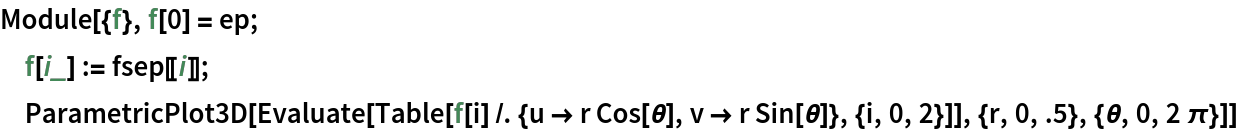Out=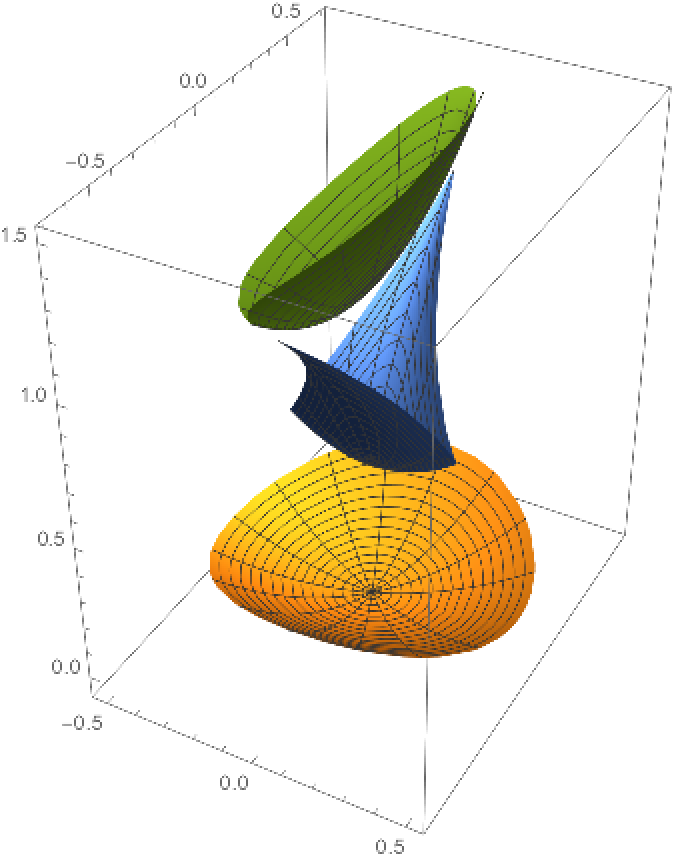### Scope (4)

A circular helicoid:

 In:=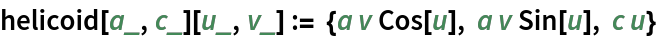Here are the two focal sets of the helicoid:

 In:=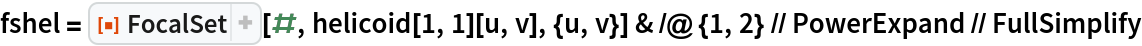Out=Plot the focal sets:

 In:=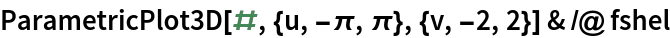Out=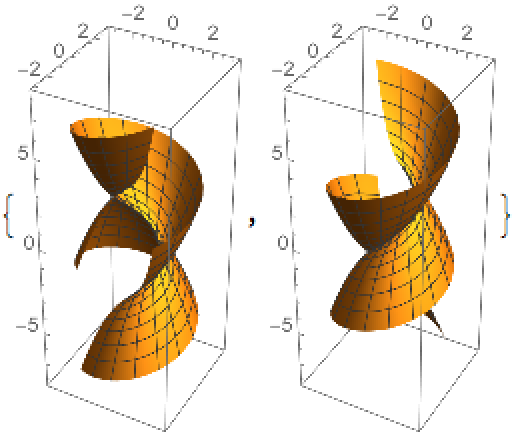Compute the focal sets of a monkey saddle:

 In:=Out=Plot of the focal sets of the monkey saddle:

 In:=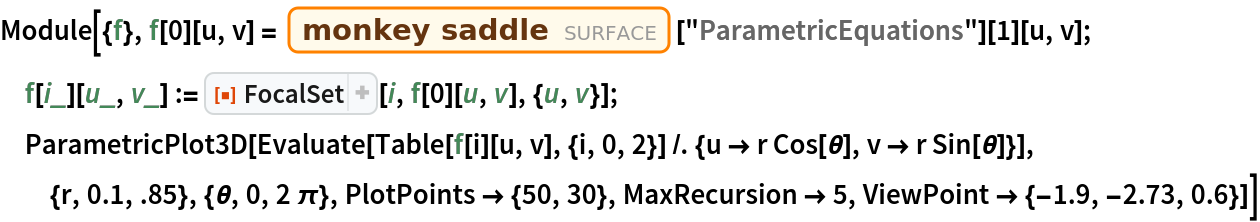Out=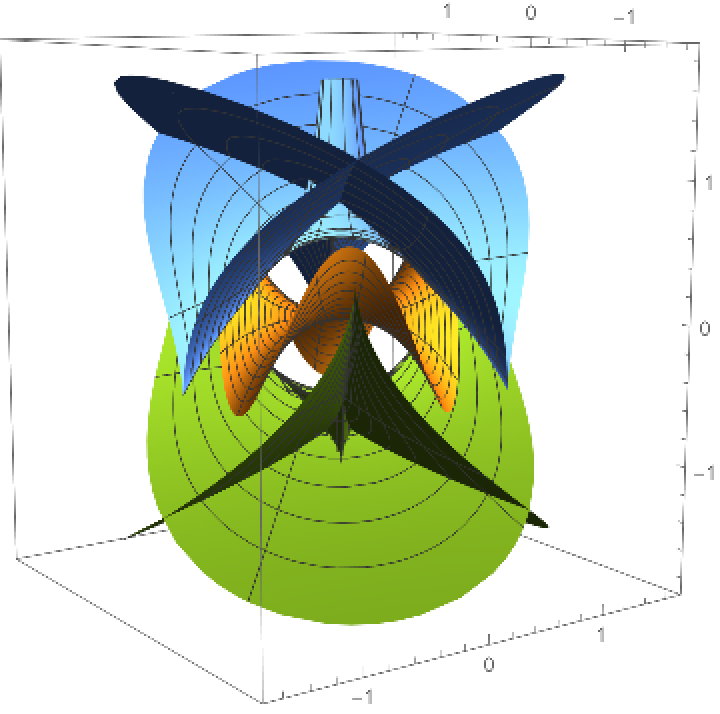It will be shown in the next example that one of the focal sets of a surface of revolution generated by a plane curve c is the surface of revolution generated by the evolute of c. First define a tractrix:

 In:=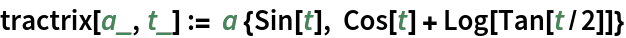Here is its evolute, as returned by the resource function EvoluteCurve:

 In:=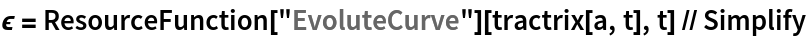Out=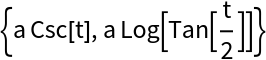The evolute of a tractrix is a catenary:

 In:=Out=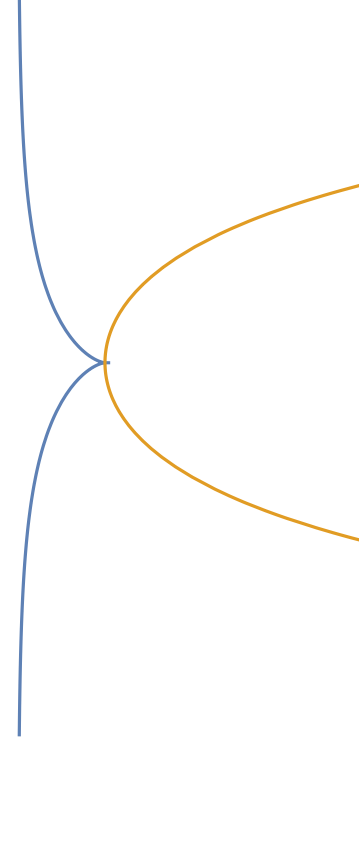Define a catenoid, i.e. the surface of revolution of a catenary:

 In:=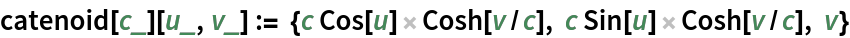Define a surface of revolution:

 In:=Compute its focal set for the first curvature:

 In:=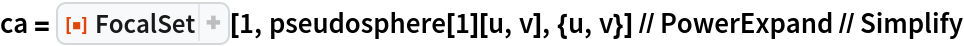Out=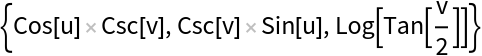Plot the pseudosphere and the catenoid (the surface of revolution of a catenary) focal sets:

 In:=Out=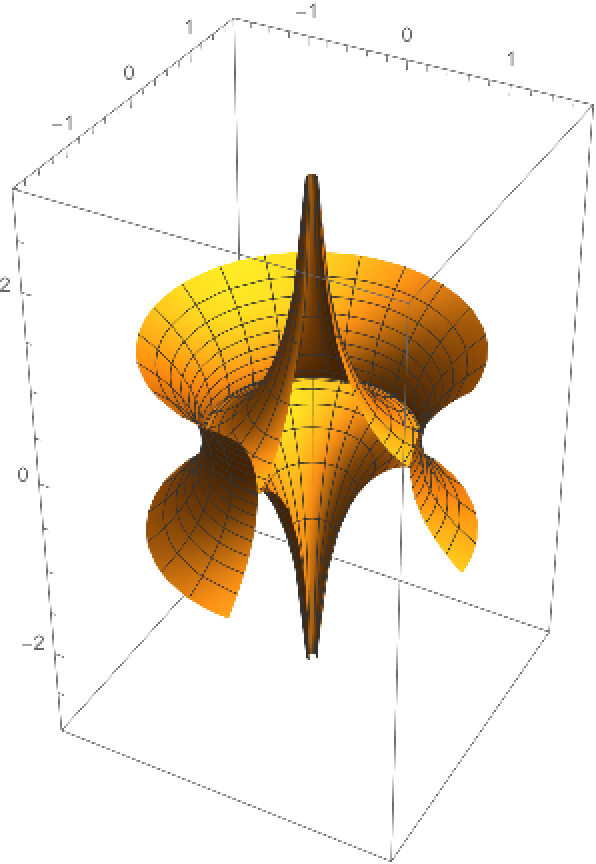Define an elliptic‐hyperbolic cyclide:

 In:=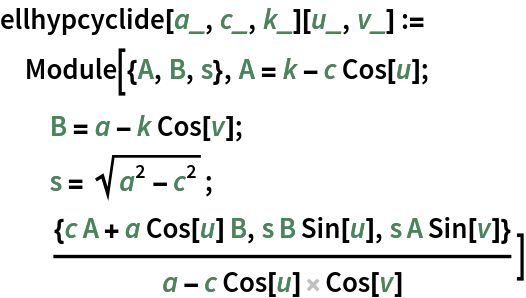Compute its focal sets:

 In:=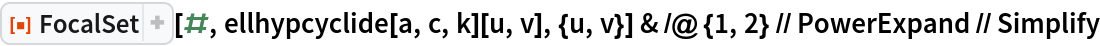Out=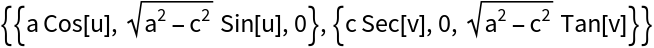Define a function to generate a cyclide together with its focal curves:

 In:=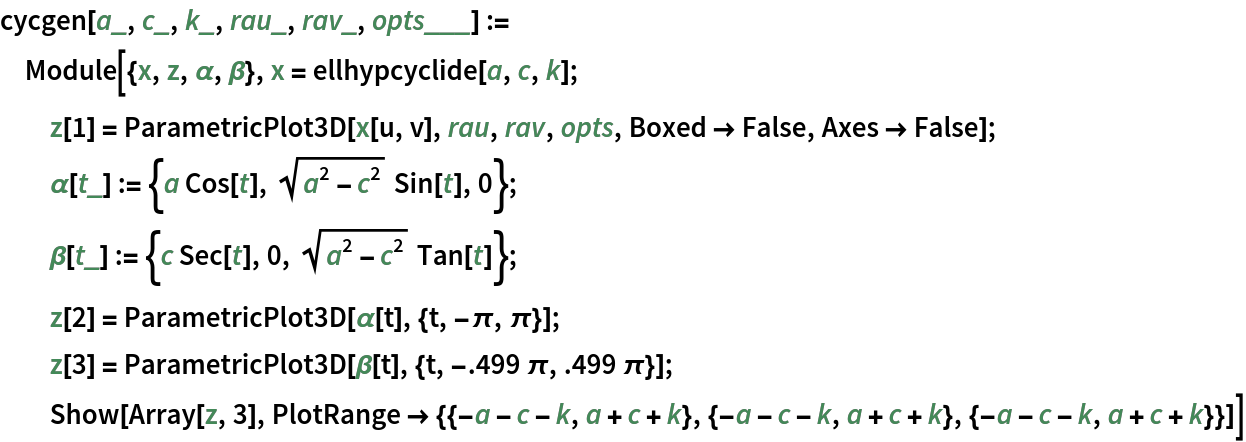The ring cyclide with its focal curves:

 In:=Out=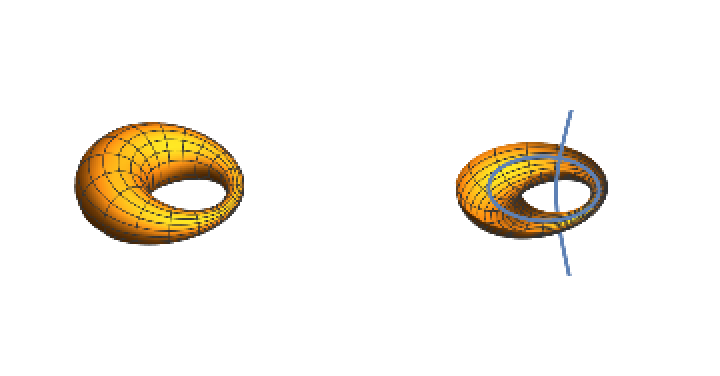The horn cyclide with its focal curves:

 In:=Out=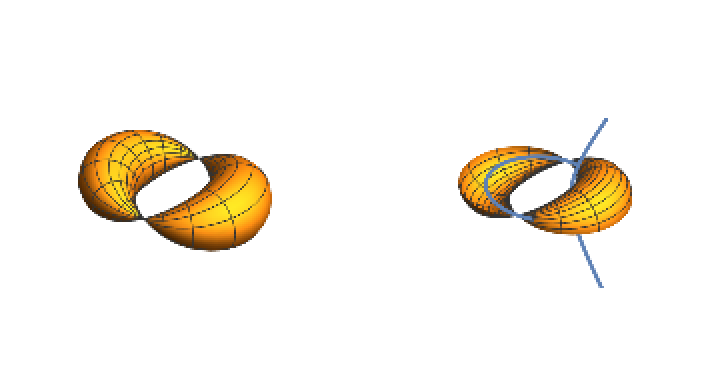The spindle cyclide with its focal curves:

 In:=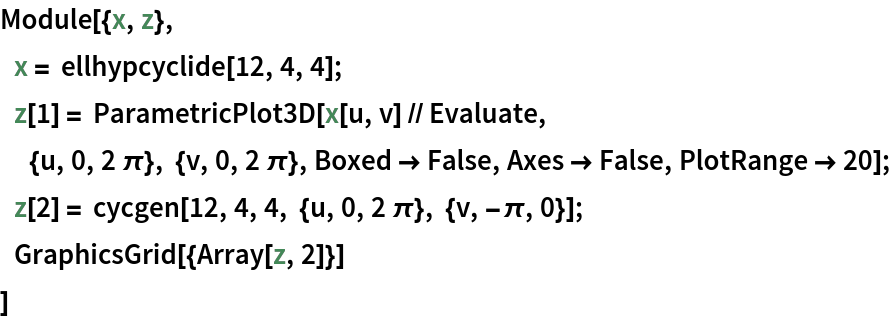Out=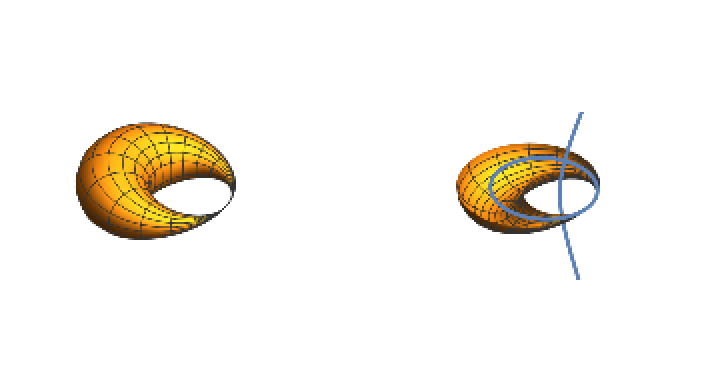Enrique Zeleny

## Version History

• 1.0.0 – 24 April 2020